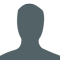## Maximum length of the sub-array whose first and last elements are same in C++Narendra Kumar

Updated on 03-Jun-2020 08:41:26

In this problem, we are given an array of characters. Our task is to create a program to print the maximum length of the subarray whose first and last elements are same in C++.Let’s take an example to understand the problem, Input − array = {‘t’, ‘u’, ‘t’, ‘o’, ‘r’, ... Read More

## Maximum modulo of all the pairs of array where arr[i] >= arr[j] in C++Narendra Kumar

Updated on 03-Jun-2020 08:40:13

In this problem, we are given an array, are of n elements. Our task is to create a program to find the maximum modulo of all pairs of array where arr[i] >= arr[j].Here, we have to find the maximum value of arr[i] % arr[j] where arr[i] >= arr[j].Let’s take an ... Read More

## Maximum number of Unique integers in Sub- Array of given sizes in C++Narendra Kumar

Updated on 03-Jun-2020 08:38:55

In this problem, we are given an array of size n and a number M. Our task is to create a program that will find the maximum number of unique integers in Sub-array of given size.Here, we will have to find the sub-array of size M that has the maximum ... Read More

## Maximum number of 3-person teams formed from two groups in C++Narendra Kumar

Updated on 03-Jun-2020 08:38:05

In this problem, we are given two integers N and M, N is the number of people in group 1, and M is the number of people in group 2. Our task is to create a program to find the maximum number of 3-person teams formed from two groups.We will ... Read More

## Maximum Path Sum in a Binary Tree in C++Narendra Kumar

Updated on 03-Jun-2020 08:35:04

In this problem, we are given a binary tree with each node containing a value. Our task is to create a program to find the maximum path sum between two leaves of a binary tree.Here, we have to find the path form one leaf node to another leaf node that ... Read More

## Maximum path sum in a triangle in C++Narendra Kumar

Updated on 03-Jun-2020 08:29:07

In this problem, we are given numbers that are in the form of a triangle. Our task is to create a program that will find the maximum path sum in a triangle.The elements are arranged starting from the 1st row with 1 one element and then next rows with an ... Read More

## Maximum path sum in an Inverted triangle in C++Narendra Kumar

Updated on 03-Jun-2020 08:27:16

In this problem, we are given numbers in the form of an inverted triangle. Our task is to create a program that will find the maximum path sum in an inverted triangle.Inverted triangle form of number is an arrangement when the first row contains n elements, second n-1, and so ... Read More

## Maximum path sum in matrix in C++Narendra Kumar

Updated on 03-Jun-2020 08:25:46

In this problem, we are given a 2D matrix of size M*N. Our task is to create a program that will find the maximum path sum in the matrix.Here, the maximum path sum in the matrix is defined as the sum of all elements for one row to the last ... Read More

## Maximum possible XOR of every element in an array with another array in C++Narendra Kumar

Updated on 03-Jun-2020 08:23:31

In this problem, we are given two arrays A and B of n elements each. Our task is to create a program to find the maximum possible XOR of every element in an array with another array.We have to compute the maximum XOR for each element of array A with ... Read More

## Maximum Primes whose sum is equal to given N in C++Narendra Kumar

Updated on 03-Jun-2020 08:21:44

In this problem, we are given a number n. Our task is to find the maximum count of primes whose sum is equal to given N.Here, we will find the maximum number of prime numbers that when added will be equal to the number.The prime number are those number which ... Read More

1 2 3 4 5 ... 20 Next